Subject: Science

#### Overview

There are two connection of loads in a circuit. They are parallel connection and series connection. This note has brief introduction on electric loads and how they can be connected to a circuit.When a wire is joined to a positive and a negative terminal of a source of electricity that completes a circuit and electrons start to flow and we get an electric current. Then we can add appliances like bulbs or bells or fans etc. to the circuit and use the current from the circuit to do some work. The appliances that use electric energy and convert it to different forms of energy are called electric loads. They pose some resistance to the flow of electricity.

### Connection of loads in a circuit:

If we have to join two or more loads in a circuit then it can be done in two ways:

1. Parallel Connection:In this connection, the loads are connected in such a way that electric current divides up among the loads but the potential difference remains the same. To achieve this, the positive terminal of all the loads (that are supposed to be in parallel connections) are connected to the same spot of the positive part of the circuit and the negative terminal of all the loads (that are supposed to be in parallel connections) are connected to the same spot of the negative terminal of the circuit. The added load work as mini circuits and work independently of each other. The brightness of the bulb is not affected due to addition or removal of bulbs in parallel connection because of same potential difference. So this type of connection is done in our house. If any one of the load fails it does not affect other loads (they work fine). In parallel connection, the reciprocal of equivalent resistance is equal to the sum of reciprocal of individual resistances i.e. $$\frac{1}{R}=\frac{1}{R_1}+\frac{1}{R_2}+\frac{1}{R_3}+...+\frac{1}{R_n}$$

2. Series Connection:In this connection, the loads are connected such a way that the electric current flowing through each resistance is equal but the potential difference is different. To achieve this, the loads are connected in series with each other (that's where the name series come from). The current flows continuously through the loads in a straight line. If one of the loads fails, the circuit becomes open and none of the loads would work. The sum of potential difference across the loads gives the total potential difference of the circuit i.e. $$V = V_1 + V_2+ V_3+...+ V_n$$

3. . The effective resistance is also the sum of the individual resistance of each load i.e. R = R1 + R2+ R+...+Rn. The brightness of the bulb is reduced when we add bulbs into the circuit because the potential difference is divided among the loads.

##### Things to remember
• There are two connection of loads in a circuit. They are parallel connection and series connection.
• The cells are connected in such a way that the potential difference of the circuit is same as the cells used in the circuit but the time of current flowing increases is called parallel connection of loads in a circuit.
• Loads are said to be connected in the series when they are daisy chained together in a single line.
• Loads in series have a common current flowing through them as the current that flows through one load must also flow through the others as it can only take one path.
• It includes every relationship which established among the people.
• There can be more than one community in a society. Community smaller than society.
• It is a network of social relationships which cannot see or touched.
• common interests and common objectives are not necessary for society.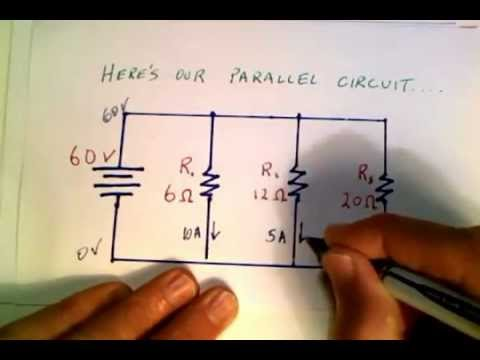##### Calculating Current in a Parallel Circuit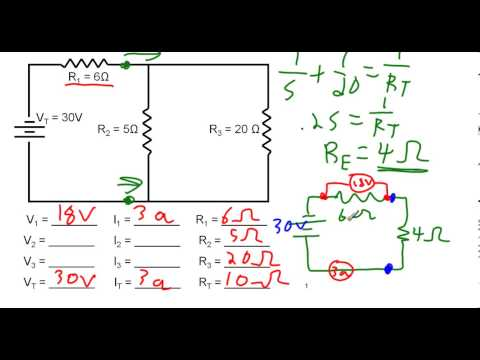##### Calculating current, total load and voltage in Complex Circuits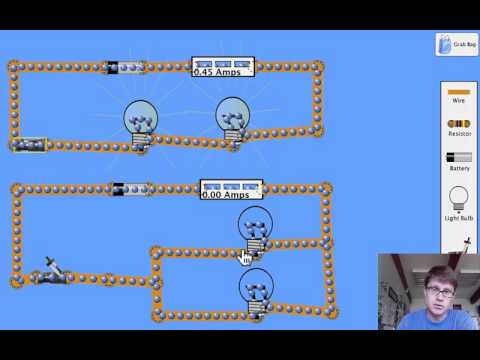##### Series and Parallel Loads in a Circuit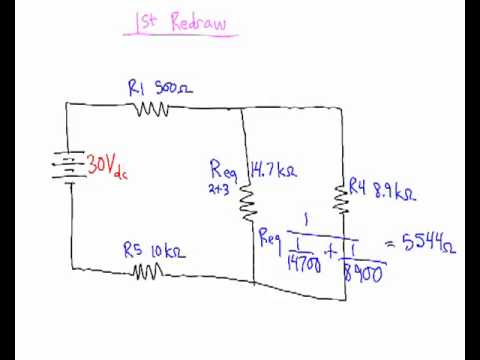##### Solving for series parallel circuits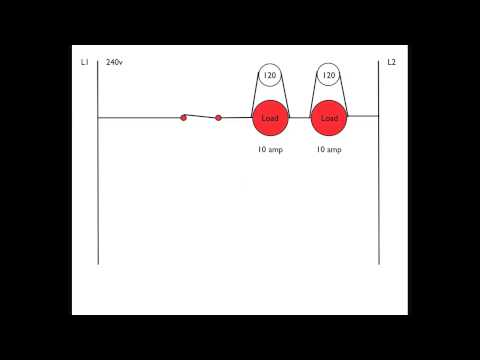##### Understanding the series load in electric circuits
The main characteristics of parallel circuit of loads are as follows: -
• Value of electric circuit in each resistor is different but value of potential difference in them is same.
• Rest resistors work, if any one of them fails to work.
• If a number of resistors are connected in parallel, the reciprocal of the equivalent resistance is equal to the sum of reciprocal of all the resistors.

The two characteristics of series circuit of load are as follows: -

• Value of electric current is same in each resistors but value of potential difference is different in them.
• The rest resistors do not work continuously, if any one of them fails to work.
• If a number of resistors are connected in series, the equivalent resistance is equal to the sum of all the resistors.
R = R1+ R2 + R3

Any two differences between parallel and series connection of resistance are as follows: -

 Series connection of load Parallel connection of load In this connection, same current flows throughout the circuit. In this connection, current will be divided into different load differently depending on their electric load. The sum of potential difference of each load gives the total potential difference. The sum of current on each load gives the total amount of current. The effective resistance 'R' of the combination is given by:R = R1+ R2 + R3……..i.e. R is more than individual resistance. The effective resistance 'R' of the combination is given by:……i.e. 'R' is less than individual resistance. In series combination, current through each resistor is same but potential difference across each resistance is different. In parallel combination,current through each resistor is different but potential difference across each resistance is same.
The energy supplied by the cell to make the flow of unit charge in an electric circuit is called its electromotive force. In short, it is written as e.m.f. It is measured in volt.
The work done against the electric field while flowing a unit charge from lower potential to higher potential is called potential difference. In short, it is written as p.d. The unit of potential difference (p.d.) is called volt. In other word:
The electrical pressure between two points which determines the flow of charge is called potential difference.

Solution,
Here,
R = 484Ω
V = 220V
I = ?
According to the formula,
V = IR0.45 A

Series combination of cells is shown in the given circuit diagram.
Here,
In the given electric circuit,
Current (I) = 0.2 A
Potential difference (V) = 1.5V + 1.5V = 3V
Power of the bulb (P) = ?
Now,
According to the formula,
P = I × V
= 3 × 0.2
= 0.6 watt
Hence, the power of the bulb is 0.6 watt.

Here,

P = 2.5 kW = 2500W (∴1kW = 1000W)

V = 200 V

I =?

We have,

P = V x I

or, 2500 = 220 x I

or, I = $\frac{2500}{220}$

= 11.36 A

Therefore, the current is 11.36 A in the circuit and a 13 A fuse is suitable for the circuit.